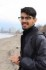# Trees in Computer Science?!?!

Learn about the tree which is a key root of knowledge in Computer Science!

In computer science, there are many ways to organize data to be used effectively. These many ways are called Data Structures. Of the many data structures, there are data structures that are hierarchical in which the data is organized into a tree-like structure. A specific tree data structure that will be taught is a Binary Search Tree.

In order to learn draw a Binary Search Tree, one must be able to learn the basic things that will help understand.

A binary search tree is similar to a family tree in a way. Before we begin, lets learn about the different terms that will be useful in understanding.

Node – a single place where data is.

Children – the data linked and below another piece of data

Root – the highest point of data

Leaf – the data at the ends

Now that you know the basics, lets learn specific topics that help understand the different forms of a tree.

Full –every node

other than the leaves has two children

Complete – every level, except possibly the last, is completely filled, and all nodes are as far left as possible

Height- the longest path from the root node to a leaf node

Depth – number of edges from the specific node from the root node

A binary search tree is a binary tree in which the nodes have a value that is greater than all values in its left subtree and smaller than all values in its right subtree.

In order to insert to a BST, you start from the root of the tree and check if the value is greater than or less than that value and if it is greater than the value, then you go to the right and check again if it is greater than or less than the value and so on and so forth until you get to the end where you add that value to the tree.

Deleting a node in BST

When deleting a node within a BST, there are many considerations to check for. The first thing to check is to see if the specific node has any children.

• If the node has 0 children
• In this case, you simply delete the node from the BST
• If the node has 1 child
• In this case, you replace the node to delete with the child
• If the node has 2 children
• In this case, you must replace the node with either the predecessor or successor
• A predecessor is the maximum value in the node’s left subtree
• A successor is the minimum value in the node’s right subtree